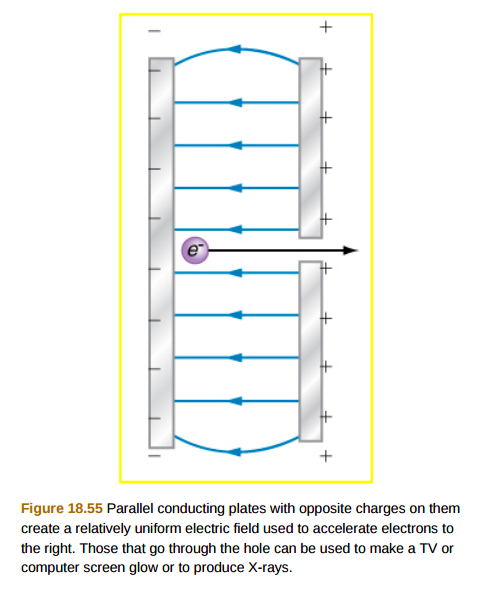# Problem: A simple and common technique for accelerating electrons is shown in Figure 18.55, where there is a uniform electric field between two plates. Electrons are released, usually a hot filament, near the negative plate, and there is a small hole in the positive plate that allows the electrons to continue moving. (a) Calculate the acceleration of the electron if the field strength is 2.50×104 N/C.(b) Explain why the electron will not be pulled back to the positive plate once it moves through the hole.

###### FREE Expert Solution

From Coulomb's law, the electrostatic force is:

$\overline{){\mathbf{F}}{\mathbf{=}}{\mathbf{q}}{\mathbf{E}}}$

Newton's second law:

$\overline{){\mathbf{F}}{\mathbf{=}}{\mathbf{m}}{\mathbf{a}}}$

(a)

From the two equations, we have, ma = qE

87% (3 ratings)###### Problem Details

A simple and common technique for accelerating electrons is shown in Figure 18.55, where there is a uniform electric field between two plates. Electrons are released, usually a hot filament, near the negative plate, and there is a small hole in the positive plate that allows the electrons to continue moving.

(a) Calculate the acceleration of the electron if the field strength is 2.50×104 N/C.

(b) Explain why the electron will not be pulled back to the positive plate once it moves through the hole.